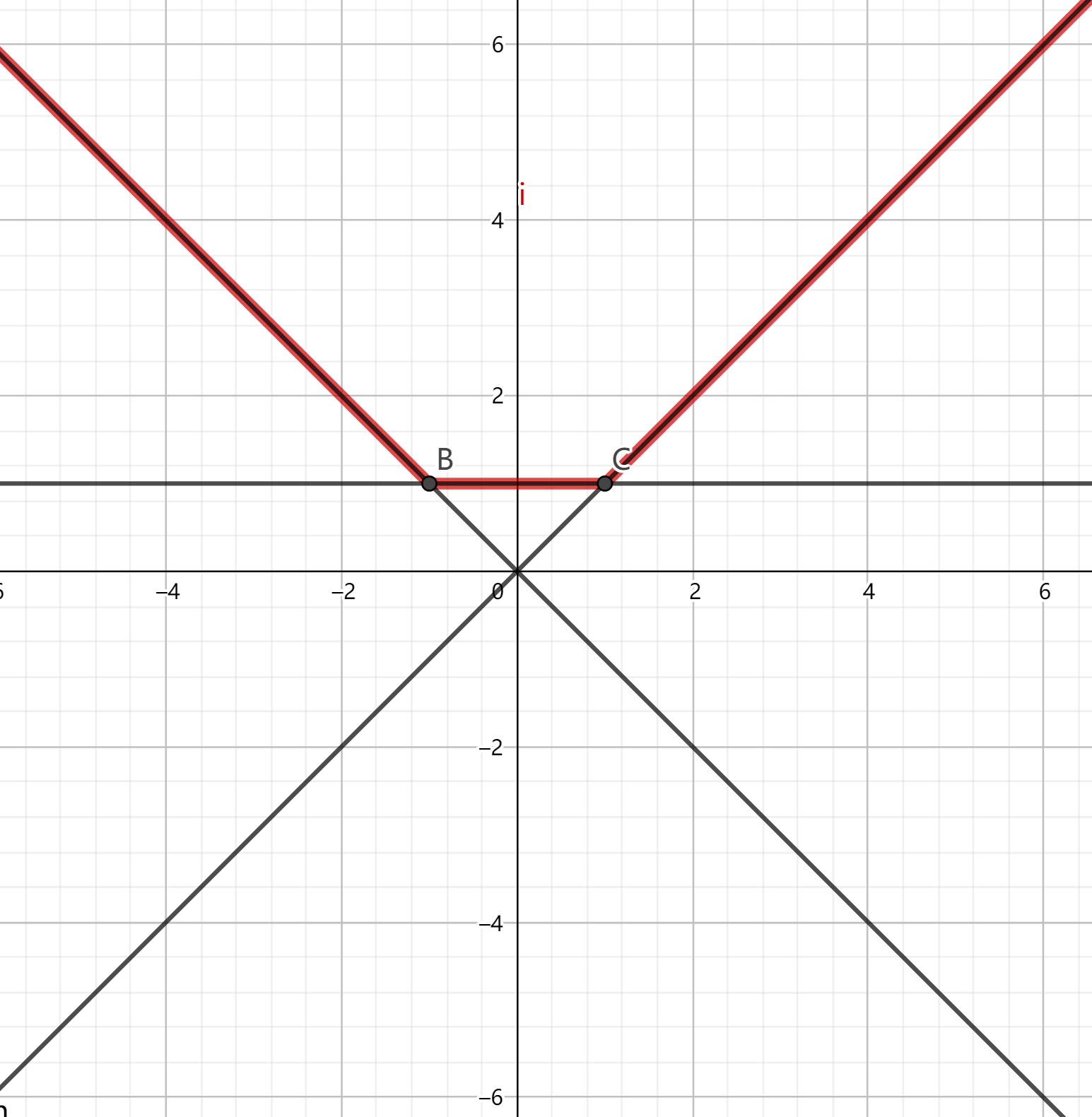# 切比雪夫距离

## 二维情况

$\max(|a-c|,|b-d|)$

$|a-c|+|b-d|=\max(|(a+b)-(c+d)|,|(a-b)-(c-d)|)$

$\max(|(a-c)+(b-d)|,|(a-c)-(b-d)|)$

## 三维情况

\begin{aligned} \ldots \leq& x+y+z &\leq \ldots\\ \ldots \leq& -x+y+z &\leq \ldots\\ \ldots \leq&x-y+z &\leq \ldots\\ \ldots \leq& x+y-z &\leq \ldots \end{aligned}

$\left\{ \begin{array}{lcr} a&=&-x+y+z\\ b&=&x-y+z\\ c&=&x+y-z \end{array} \right.$

\begin{aligned} \ldots \leq& a+b+c &\leq \ldots\\ \ldots \leq& a &\leq \ldots\\ \ldots \leq& b &\leq \ldots\\ \ldots \leq& c &\leq \ldots \end{aligned}

# 斜线转坐标轴平行线

$-(a-x_0)\leq b-y_0\leq a-x_0\\ \Rightarrow \left\{ \begin{array}{ccc} y_0+x_0&\leq &b+a\\ y_0-x_0&\geq &b-a \end{array} \right.$

$y_0-(x-x_0)\frac{p}{q}\leq y\leq y_0+(x-x_0)\frac{p}{q}$

$y_0q-(xp-x_0p)\leq yq\leq y_0q+(xp-x_0p)$

# 求三角形内心坐标

$\exp(ix)=\cos(x)+i\sin(x)$

$\exp(i\frac{\varphi_1+\varphi_2}{2})-\exp(i\frac{\varphi_1+\varphi_3}{2})+\exp(i\frac{\varphi_2+\varphi_3}{2})$

# 曼哈顿距离最小生成树# 凸包问题

• $\sum_{i=1}^na_ix_i\geq A$
• $\sum_{i=1}^nb_ix_i\geq B$

• $\sum_{i=1}^na_ix_i\leq A$
• $\sum_{i=1}^nb_ix_i\leq B$

# Delaunay triangulation问题Delaunay triangulation的一些用处：

• 三角形的边最多有$3n-6$条。
• 欧几里德最小生成树是三角化后所有三角形的边的子集。
• 所有三角形的外切圆内（圆周上不算），都不会包含其余顶点。
• 对于任意顶点，距离它的最近点一定在三角化后与它拥有公共边。

# 多边形相交

## 两个多边形相交

• 一个多边形被包含在另外一个多边形内部
• 两个多边形存在两条边有交点

## 两个等速旋转的多边形是否相交

1. $A$的某个顶点和$B$的某条边有交点
2. $B$的某个顶点和$A$的某条边有交点

# 凸包技巧

• 向集合插入一个一阶多项式。
• 询问集合中所有一阶多项式在某个特定$x=x_0$值处的值的最大值。

$f(x)=\max_{y\in Y}y(x)$# 单调增斜率优化

$f(i)=\Bigg\{ \begin{array}{ccc} 0&,i=0\\ \min\{h(j)-s(i)s(j)|0\leq j <i\}&,i\ge 1 \end{array}$

$r(j,i) \leq r(k,i)\\ \Rightarrow h(j)-s(i)s(j) \leq h(k)-s(i)s(k)\\ \Rightarrow h(j)-h(k) \leq s(i)(s(j)-s(k))\\ \Rightarrow \frac{h(j)-h(k)}{s(j)-s(k)}\leq s(i)$

$r(j,i) \leq r(k,i)\\ \Rightarrow \frac{Y(j)-Y(k)}{X(j)-X(k)} \leq s(i)$

SRM787 div1第一题。